f
Neutrium

# Reynolds Number

Index of Dimensionless Numbers

Dimensionless numbers play an important role in analysing fluid dynamics and heat and mass transfer problems. They provide a method by which complex phenomena can be characterised, often by way of a simple, single number comparison. This article provides a summary of dimensionless numbers and the formulae used to calculate them.

Dimensionless Numbers and Dimensional Analysis

Dimensionless numbers play an important role in analysing fluid dynamics and heat and mass transfer problems. They provide a method by which complex phenomena can be characterised, often by way of a simple, single number comparison. This article provides a brief overview of the derivation and use of dimensionless numbers.

Sherwood NumberThe Sherwood number is a dimensionless number that represents the ratio of convective mass transfer to the rate of diffusive mass transport and is used in the analysis of mass transfer systems such as liquid-liquid extraction. This article describes the Sherwood number and typical formulations.

Schmidt NumberThe Schmidt number is a dimensionless number that describes the ratio of momentum diffusivity to mass diffusivity that is commonly used in analysis of mass transfer systems. This article describes the Schmidt Number and typical formulations.

Pressure Drop Through a Packed Bed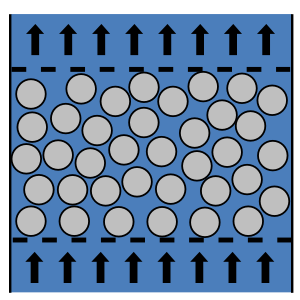As fluid flows through a packed bed it experiences a pressure loss due to friction. This article describes the use of the Carman-Kozeny and Ergun equations for the calculation of pressure drop through a randomly packed bed of spheres.

Terminal Velocity of Particles for Gravity Separation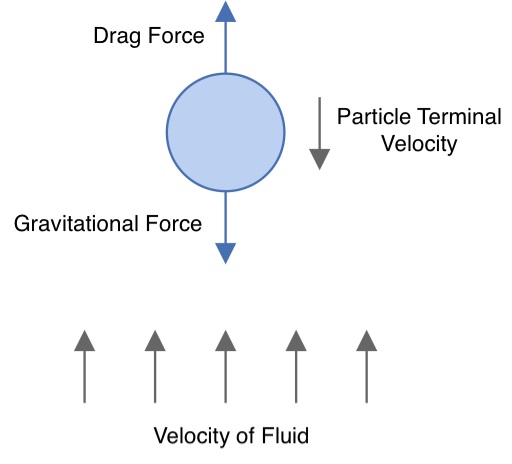There are many operations in which two phases must be separated. These separations may be gas-liquid, gas-solid, liquid-liquid or liquid-solid, with several factors such as relative densities, gravity, fluid velocities and the shape of particles and/or droplets influence the phase separability. In this article we present the fundamentals of these separations and provide the Stoke’s, Intermediate and Newton’s formulae for calculating the terminal velocities of settling particles to analyse separation systems.

Packed Bed Reynolds NumberThe packed bed Reynolds number is dimensionless and describes the ratio of inertial to viscous forces for fluid flow through a packed bed. It may be used to calculate the pressure drop though a packed bed via the Ergun equation or identify the boundaries of flow regimes (laminar, transitional and turbulent) in a packed bed. This article will show you how to calculate and interpret the packed bed Reynolds number.

Friction Factor for Flow in Coils and Curved Pipe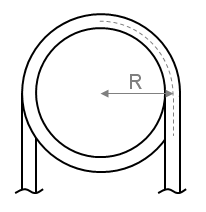In order to determine the pressure drop in a pipe or coil the friction factor must first be calculated. This article presents the equations which may be used to determine the friction factor in coils and curved pipe.

Pressure Loss in Pipe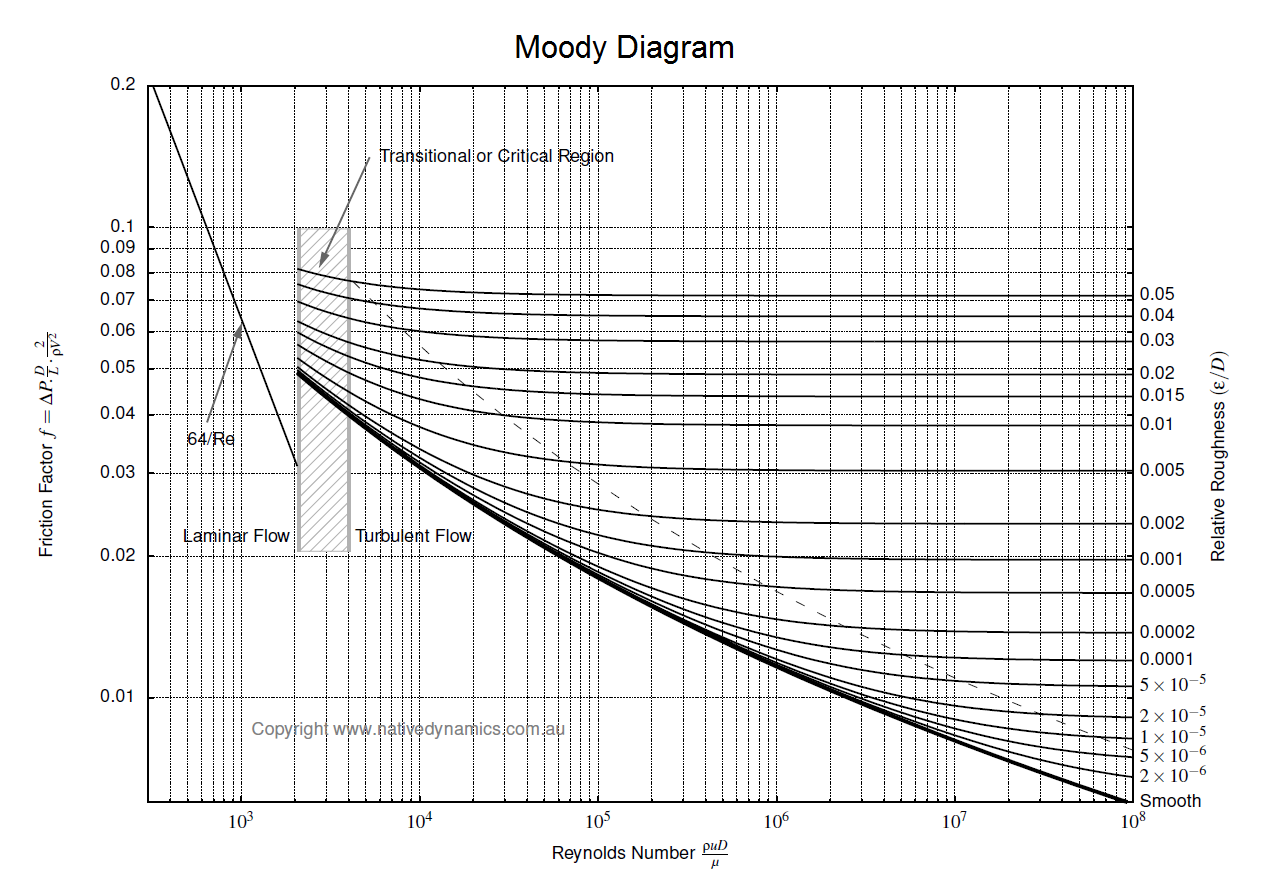To determine the pressure loss or flow rate through pipe knowledge of the friction between the fluid and the pipe is required. This article describes how to incorporate friction into pressure loss or fluid flow calculations. It also outlines several methods for determining the Darcy friction factor for rough and smooth pipes in both the turbulent and laminar flow regime. Finally this article discusses which correlation for pressure loss in pipe is the most appropriate.

Reynolds Number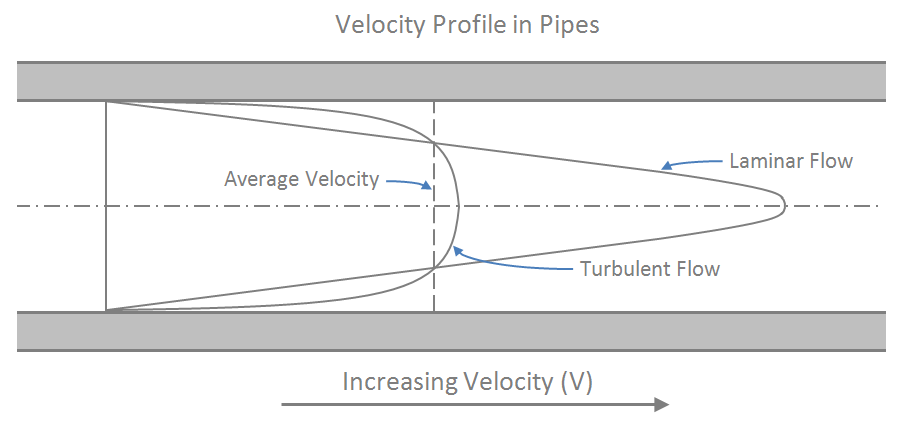The Reynolds number is dimensionless and describes the ratio of inertial forces to viscous forces in a flowing fluid. It is used in many fluid flow correlations and is used to describe the boundaries of fluid flow regimes (laminar, transitional and turbulent). This article will show you how to calculate and interpret the Reynolds number.